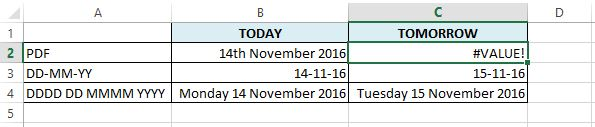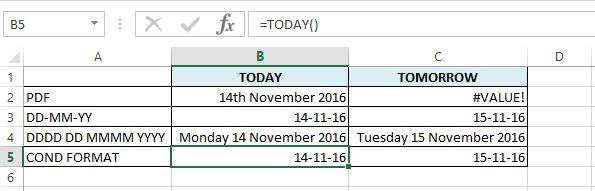Excel Format dates to include st, nd,rd and thIn an eBook “Date Functions”, I showed you a formula that would include st, nd, rd and th to a day number, so 14 November would display as 14th November.  I have since been asked by some people if it is possible to format dates to include st, nd, rd and th.

The answer is yes, you can apply a format, but a formula is needed to do this. Confused?

Let’s start from the start, with what I covered in the eBook.

Formula to include st, nd, rd and th in Dates

One of the last section of the Date Functions eBook gave you a formula that would add st, nd, rd and th to a day number in a date.

Based on today’s date (November 14, 2016), the result of this formula would be 14th November 2016.

The formula is =DAY(TODAY())&LOOKUP(DAY(TODAY()),{1,2,3,4,21,22,23,24,31;”st”,”nd”,”rd”,”th”,”st”,”nd”,”rd”,”th”,”st”})&TEXT(TODAY(),” mmmm yyy”).

In this post, I won’t go through this formula in detail, you can refer to the eBook for that.

The result of this formula creates a text string of 14th November 2016, this result cannot be used in a simple formula.

In the image below, column B gives today’s date and column C gives tomorrow’s date by adding 1 to today’s date.Working from the bottom:

• Row 4 gives the date in the custom format DDDD DD MMMM YYYY, B4 uses the TODAY() function to get today’s date and C4 adds 1 to that to give tomorrow’s date.
• Row 3 uses the custom format DD-MM-YY and again, B3 uses the TODAY() function and C3 adds one to this.
• Row 2 uses the formula from the eBook (PDF format) to display today’s date with the th.  As you can see, adding 1 to today’s date does not work here because 1 is being added to a text string.

This is why I was asked how to format dates to include st, nd, rd and th.

Format dates to include st, nd, rd and th

To format dates to include st, nd, rd and th we are going to use conditional formatting.

We will apply a formula to the conditional formatting that will select what number format to use.

I’ve added another row to our last image.

In this row we will set up the conditonal formatting.

B5 has TODAY() function and C5 adds 1 to this to give tomorrow’s date.We will now set up the conditional formats to add the letters to our date.

This can be broken down into 2 main sections.

1 Create Custom Number Formats

• Highlight the cells to be formatted (B5 to C5)
• Right-click
• Select Format cells
• On the Number tab, go to the “Custom” section
• Set the format as d”st” Mmmm yyy (day number in number format, followed by st in quotes with no space between, then space and the full month name and finally the year including 20).• Repeat again with “nd” instead of “st”
• Then again with “rd”
• Then one last time with “th”.

We have set up the number formats, but now we need to tell Excel when to use each format.2 Conditional Formatting

• Click on cell B5
• On the Home ribbon, go to Conditional Formatting
• Create a New Rule
• Select the rule type “Use a formula to determine which cells to format”.
• Enter the formula “=OR(DAY(B5)=1,DAY(B5)=11,DAY(B5)=21,DAY(B5)=31)
• Set the format to the custom number format we created earlier with “st”.  If the day number in our cell is 1, 11, 21 or 31, then Excel will apply the number format with “st”.• Create another rule with the rule type to “Use a formula to determine which cells to format”.
• Enter the formula “=OR(DAY(B5)=2,DAY(B5)=22)
• Set the format to the custom number format we created earlier with “nd”.  If the day number in our cell is 2 or 22, then Excel will apply the number format with “nd”.
• Repeat creating a new rule again.
• This time enter the formula “=OR(DAY(B5)=3,DAY(B5)=23)
• Set the format to the custom number format we created with “rd”.  If the day number is 3 or 23, then Excel will apply the number format with “rd”.
• Create one final rule.
• This time enter the formula “=OR(AND(DAY(B5)>=4,DAY(B5)<=20),AND(DAY(B5)>=24,DAY(B5)<=30))
• Set the format to the custom number format we created with “th”.  If the day number is between 4 and 20 (inclusive) or the day number is between 24 and 30 (inclusive) then apply the number format with “th”.• Apply these formatting rules to our cell.
• You can see that Excel is applying the “th” to our date because of the set rules.• You can see that the formula in C5 is still working.  We did not change the date in B5 to be a string, we only changed the format of the cell to show the relevant characters.
• Copy B5
• Paste the format only to C5.
• Change the dates to check that the formatting rules work.